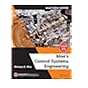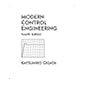Dr. S. R. Lasker Library Online Catalogue

Normal view

# Modern control engineering / Katsuhiko Ogata.

Material type:TextLanguage: English Publication details: c2002. Edition: 4th edDescription: xi, 964 p. : ill. ; 24 cmISBN: 0130609072; 9780130609076Subject(s): Automatic control | Control theoryDDC classification: 629.8 LOC classification: TJ213 | .O28 2002Online resources: WorldCat details | Ebook Fulltext
Contents:
(NOTE: Each chapter begins with Introduction and concludes with Example Problems and Solutions and Problems.) 1. Introduction to Control Systems. Examples of Control Systems. Closed-Loop Control versus Open-Loop Control. Outline of the Book. 2. The Laplace Transform. Review of Complex Variables and Complex Functions. Laplace Transformation. Laplace Transform Theorems. Inverse Laplace Transformation. Partial-Fraction Expansion with MATLAB. Solving Linear, Time-Invariant, Differential Equations. 3. Mathematical Modeling of Dynamic Systems. Transfer Function and Impulse-Response Function. Automatic Control Systems. Modeling in State Space. State-Space Representation of Dynamic Systems. Transformation of Mathematical Models with MATLAB. Mechanical Systems. Electrical and Electronic Systems. Signal Flow Graphs. Linearization of Nonlinear Mathematical Models. 4. Mathematical Modeling of Fluid Systems and Thermal Systems. Liquid-Level Systems. Pneumatic Systems. Hydraulic Systems. Thermal Systems. 5. Transient and Steady-State Response Analyses. First-Order Systems. Second-Order Systems. Higher-Order Systems. Transient-Response Analysis with MATLAB. An Example Problem Solved with MATLAB. Routh's Stability Criterion. Effects of Integral and Derivative Control Actions on System Performance. Steady-State Errors in Unity-Feedback Control Systems. 6. Root-Locus Analysis. Root-Locus Plots. Summary of General Rules for Constructing Root Loci. Root-Locus Plots with MATLAB. Positive-Feedback Systems. Conditionally Stable Systems. Root Loci for Systems with Transport Lag. 7. Control Systems Design by the Root-Locus Method. Preliminary Design Considerations. Lead Compensation. Lag Compensation. Lag-Lead Compensation. Parallel Compensation. 8. Frequency-Response Analysis. Bode Diagrams. Plotting Bode Diagrams with MATLAB. Polar Plots. Drawing Nyquist Plots with MATLAB. Log-Magnitude-versus-Phase Plots. Nyquist Stability Criterion. Stability Analysis. Relative Stability. Closed-Loop Frequency Response of Unity-Feedback Systems. Experimental Determination of Transfer Functions. 9. Control Systems Design by Frequency Response. Lead Compensation. Lag Compensation. Lag-Lead Compensation. Concluding Comments. 10. PID Controls and Two-Degrees-of-Freedom Control Systems. Tuning Rules for PID Controllers. Computational Approach to Obtain Optimal Sets of Parameter Values. Modifications of PID Control Schemes. Two-Degrees-of-Freedom Control. Zero-Placement Approach to Improve Response Characteristics. 11. Analysis of Control Systems in State Space. State-Space Representations of Transfer-Function Systems. Transformation of System Models with MATLAB. Solving the Time-Invariant State Equation. Some Useful Results in Vector-Matrix Analysis. Controllability. Observability. 12. Design of Control Systems in State Space. Pole Placement. Solving Pole-Placement Problems with MATLAB. Design of Servo Systems. State Observers. Design of Regulator Systems with Observers. Design of Control Systems with Observers. Quadratic Optimal Regulator Systems. References. Index. TOC
Summary: For senior/graduate-level first courses in Control Theory in departments of Mechanical, Electrical, Aerospace, and Chemical Engineering. This comprehensive treatment of the analysis and design of continuous-time control systems provides a gradual development of control theory - and shows how to solve all computational problems with MATLAB.
Tags from this library: No tags from this library for this title.
Star ratingsAverage rating: 0.0 (0 votes)
Holdings
Item type Current library Collection Call number Copy number Status Date due Barcode Item holds Course reservesE-Book
E-book
Non-fiction 629.8 OGM 2006 (Browse shelf(Opens below)) Not for loanText
Reserve Section
Non-fiction 629.8 OGM 2006 (Browse shelf(Opens below)) C-1 Not For Loan 20417Text
Circulation Section
Non-fiction 629.8 OGM 2006 (Browse shelf(Opens below)) C-2 Available 20418Text
Circulation Section
Non-fiction 629.8 OGM 2006 (Browse shelf(Opens below)) C-3 Available 20961Text
Circulation Section
Non-fiction 629.8 OGM 2006 (Browse shelf(Opens below)) C-4 Available 20962Text
Circulation Section
Non-fiction 629.8 OGM 2006 (Browse shelf(Opens below)) C-5 Available 20963Text
Circulation Section
Non-fiction 629.8 OGM 2006 (Browse shelf(Opens below)) C-6 Available 20964
Total holds: 0
##### Browsing EWU Library shelves, Shelving location: Circulation Section Close shelf browser (Hides shelf browser)629.8 NIN 2018 Nise's control systems engineering / 629.8 NIN 2018 Nise's control systems engineering / 629.8 OGM 2006 Modern control engineering / 629.8 OGM 2006 Modern control engineering / 629.8 OGM 2006 Modern control engineering / 629.8 OGM 2006 Modern control engineering / 629.8 OGM 2006 Modern control engineering /

Includes bibliographical references (952-953) and index.

Includes bibliographical references and index.

(NOTE: Each chapter begins with Introduction and concludes with Example Problems and Solutions and Problems.) 1. Introduction to Control Systems. Examples of Control Systems. Closed-Loop Control versus Open-Loop Control. Outline of the Book. 2. The Laplace Transform. Review of Complex Variables and Complex Functions. Laplace Transformation. Laplace Transform Theorems. Inverse Laplace Transformation. Partial-Fraction Expansion with MATLAB. Solving Linear, Time-Invariant, Differential Equations. 3. Mathematical Modeling of Dynamic Systems. Transfer Function and Impulse-Response Function. Automatic Control Systems. Modeling in State Space. State-Space Representation of Dynamic Systems. Transformation of Mathematical Models with MATLAB. Mechanical Systems. Electrical and Electronic Systems. Signal Flow Graphs. Linearization of Nonlinear Mathematical Models. 4. Mathematical Modeling of Fluid Systems and Thermal Systems. Liquid-Level Systems. Pneumatic Systems. Hydraulic Systems. Thermal Systems. 5. Transient and Steady-State Response Analyses. First-Order Systems. Second-Order Systems. Higher-Order Systems. Transient-Response Analysis with MATLAB. An Example Problem Solved with MATLAB. Routh's Stability Criterion. Effects of Integral and Derivative Control Actions on System Performance. Steady-State Errors in Unity-Feedback Control Systems. 6. Root-Locus Analysis. Root-Locus Plots. Summary of General Rules for Constructing Root Loci. Root-Locus Plots with MATLAB. Positive-Feedback Systems. Conditionally Stable Systems. Root Loci for Systems with Transport Lag. 7. Control Systems Design by the Root-Locus Method. Preliminary Design Considerations. Lead Compensation. Lag Compensation. Lag-Lead Compensation. Parallel Compensation. 8. Frequency-Response Analysis. Bode Diagrams. Plotting Bode Diagrams with MATLAB. Polar Plots. Drawing Nyquist Plots with MATLAB. Log-Magnitude-versus-Phase Plots. Nyquist Stability Criterion. Stability Analysis. Relative Stability. Closed-Loop Frequency Response of Unity-Feedback Systems. Experimental Determination of Transfer Functions. 9. Control Systems Design by Frequency Response. Lead Compensation. Lag Compensation. Lag-Lead Compensation. Concluding Comments. 10. PID Controls and Two-Degrees-of-Freedom Control Systems. Tuning Rules for PID Controllers. Computational Approach to Obtain Optimal Sets of Parameter Values. Modifications of PID Control Schemes. Two-Degrees-of-Freedom Control. Zero-Placement Approach to Improve Response Characteristics. 11. Analysis of Control Systems in State Space. State-Space Representations of Transfer-Function Systems. Transformation of System Models with MATLAB. Solving the Time-Invariant State Equation. Some Useful Results in Vector-Matrix Analysis. Controllability. Observability. 12. Design of Control Systems in State Space. Pole Placement. Solving Pole-Placement Problems with MATLAB. Design of Servo Systems. State Observers. Design of Regulator Systems with Observers. Design of Control Systems with Observers. Quadratic Optimal Regulator Systems. References. Index. TOC

For senior/graduate-level first courses in Control Theory in departments of Mechanical, Electrical, Aerospace, and Chemical Engineering. This comprehensive treatment of the analysis and design of continuous-time control systems provides a gradual development of control theory - and shows how to solve all computational problems with MATLAB.

EEE

Shaharima Parvin

There are no comments on this title.